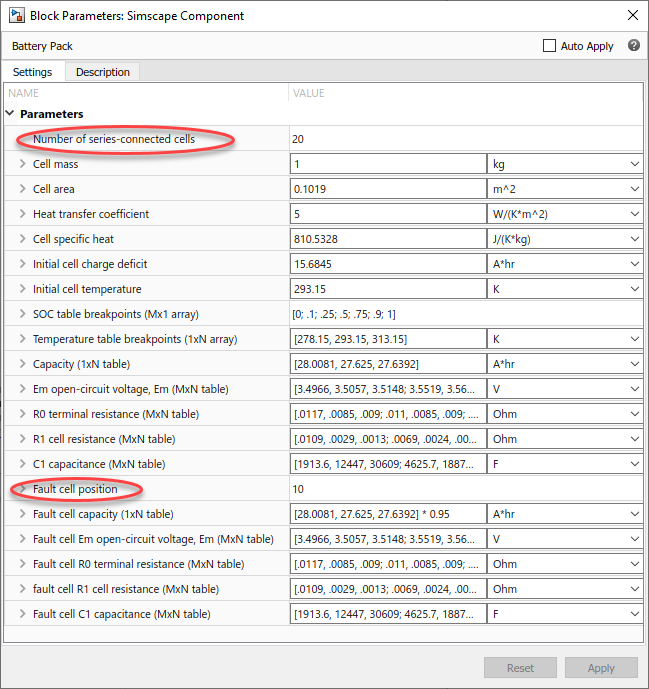## Case Study — Battery Pack with Fault Using Arrays

### Overview of the Model

This case study explains how you can use component arrays to model a battery pack consisting of multiple series-connected cells. It also shows how you can introduce a fault into one of the cells to see the impact on battery performance and cell temperatures. Both the number of cells and the position of the faulted cell are the top-level component parameters modifiable by the block user.

The case study is based on the Lithium-Ion Battery Pack with Fault Using Arrays example. To open the example model, type `ssc_lithium_battery_arrays` in the MATLAB® Command Window.

The Battery Pack block is a composite component modeling an array of battery cells. The source files for this example are in the following package folder:

`matlabroot/toolbox/physmod/simscape/simscapedemos/+BatteryPack`

where `matlabroot` is the MATLAB root directory on your machine, as returned by entering

```matlabroot ```

in the MATLAB Command Window.

The `+BatteryPack` package contains the following files:

• `battery_cell.ssc` — Component file representing the individual battery cell. The source for this component is generated using `subsystem2ssc` from the Lithium Cell 1RC subsystem in the Lithium Battery Cell - One RC-Branch Equivalent Circuit example.

• `battery_pack.ssc` — Composite component that models the battery pack as an array of `battery_cell` components.

```component battery_pack % Battery Pack % This block models a scalable battery pack with faults using arrays. % Copyright 2019 The MathWorks, Inc. parameters Ncells = 20; % Number of series-connected cells cell_mass = {1, 'kg'}; % Cell mass cell_area = {0.1019, 'm^2'}; % Cell area h_conv = {5, 'W/(m^2 * K)'}; % Heat transfer coefficient cell_Cp_heat = {810.5328, 'J/(kg*K)'}; %Cell specific heat Qe_init = {15.6845, 'hr*A'}; %Initial cell charge deficit T_init = {293.15, 'K'}; % Initial cell temperature SOC_LUT = [0; .1; .25; .5; .75; .9; 1]; %SOC table breakpoints (Mx1 array) Temperature_LUT = {[278.15, 293.15, 313.15], 'K'}; %Temperature table breakpoints (1xN array) Capacity_LUT = {[28.0081, 27.625, 27.6392], 'hr*A'}; %Capacity (1xN table) Em_LUT = {[3.4966, 3.5057, 3.5148; 3.5519, 3.566, 3.5653; 3.6183, 3.6337, 3.6402; 3.7066, 3.7127, 3.7213; 3.9131, 3.9259, 3.9376; 4.0748, 4.0777, 4.0821; 4.1923, 4.1928, 4.193], 'V'}; %Em open-circuit voltage, Em (MxN table) R0_LUT = {[.0117, .0085, .009; .011, .0085, .009; .0114, .0087, .0092; .0107, .0082, .0088; .0107, .0083, .0091; .0113, .0085, .0089; .0116, .0085, .0089], 'Ohm'}; %R0 terminal resistance (MxN table) R1_LUT = {[.0109, .0029, .0013; .0069, .0024, .0012; .0047, .0026, .0013; .0034, .0016, .001; .0033, .0023, .0014; .0033, .0018, .0011; .0028, .0017, .0011], 'Ohm'}; %R1 cell resistance (MxN table) C1_LUT = {[1913.6, 12447, 30609; 4625.7, 18872, 32995; 23306, 40764, 47535; 10736, 18721, 26325; 18036, 33630, 48274; 12251, 18360, 26839; 9022.9, 23394, 30606], 'F'}; %C1 capacitance (MxN table) % Fault cell fault_cell_position = 10; %Fault cell position fault_cell_Capacity_LUT = {[28.0081, 27.625, 27.6392]*0.95, 'hr*A'}; %Fault cell capacity (1xN table) fault_cell_Em_LUT = {[3.4966, 3.5057, 3.5148; 3.5519, 3.566, 3.5653; 3.6183, 3.6337, 3.6402; 3.7066, 3.7127, 3.7213; 3.9131, 3.9259, 3.9376; 4.0748, 4.0777, 4.0821; 4.1923, 4.1928, 4.193]*0.90, 'V'}; %Fault cell Em open-circuit voltage, Em (MxN table) fault_cell_R0_LUT = {[.0117, .0085, .009; .011, .0085, .009; .0114, .0087, .0092; .0107, .0082, .0088; .0107, .0083, .0091; .0113, .0085, .0089; .0116, .0085, .0089]*5, 'Ohm'}; % Fault cell R0 terminal resistance (MxN table) fault_cell_R1_LUT = {[.0109, .0029, .0013; .0069, .0024, .0012; .0047, .0026, .0013; .0034, .0016, .001; .0033, .0023, .0014; .0033, .0018, .0011; .0028, .0017, .0011]*5, 'Ohm'}; % fault cell R1 cell resistance (MxN table) fault_cell_C1_LUT = {[1913.6, 12447, 30609; 4625.7, 18872, 32995; 23306, 40764, 47535; 10736, 18721, 26325; 18036, 33630, 48274; 12251, 18360, 26839; 9022.9, 23394, 30606]*0.95, 'F'}; % Fault cell C1 capacitance (MxN table) end nodes p = foundation.electrical.electrical; % +:top n = foundation.electrical.electrical; % -:bottom H = foundation.thermal.thermal; % H:bottom end variables(Access=protected) T = {ones(1,Ncells),'K'}; SOC = ones(1,Ncells); end outputs m = {ones(1,Ncells),'K'}; % m:top end for i =1:Ncells components(ExternalAccess=none) battery_cell(i) = BatteryPack.battery_cell(cell_mass=cell_mass,cell_Cp_heat=cell_Cp_heat,... C1_LUT=(if i==fault_cell_position,fault_cell_C1_LUT;else C1_LUT; end),... SOC_LUT=SOC_LUT,Temperature_LUT=Temperature_LUT,... Capacity_LUT=(if i==fault_cell_position,fault_cell_Capacity_LUT;else Capacity_LUT; end),... Em_LUT=(if i==fault_cell_position,fault_cell_Em_LUT;else Em_LUT; end),Qe_init=Qe_init,... R0_LUT=(if i==fault_cell_position,fault_cell_R0_LUT;else R0_LUT; end),... R1_LUT=(if i==fault_cell_position,fault_cell_R1_LUT;else R1_LUT; end),T_init.value=T_init); convection(i) = foundation.thermal.elements.convection(area=cell_area,heat_tr_coeff=h_conv); end connections connect(battery_cell(i).H,convection(i).B); connect(H,convection(i).A); end end connections connect(battery_cell(1).p,p); connect(battery_cell(Ncells).n,n); end equations assert(mod(Ncells, 1) == 0 && Ncells > 0, 'Number of series-connected cells must be a positive integer'); assert(mod(fault_cell_position, 1) == 0 && fault_cell_position > 0 && fault_cell_position <= Ncells , 'Fault cell position must be a positive integer less than or equal to Number of series-connected cells'); T == [battery_cell.T_init]; SOC == [battery_cell.SOC]; m == T; end for i=1:Ncells-1 components(ExternalAccess=none) conduction(i) = foundation.thermal.elements.conduction(area={1e-3,'m^2'},... th_cond={200,'W/(m*K)'}); end connections connect(battery_cell(i+1).p,battery_cell(i).n); connect(battery_cell(i).H,conduction(i).A); connect(battery_cell(i+1).H,conduction(i).B); end end end ```

This schematic represents the equivalent circuit for the composite component.The composite component has two electrical nodes, `p` and `n`, and one thermal node, `H`:

```nodes p = foundation.electrical.electrical; % +:top n = foundation.electrical.electrical; % -:bottom H = foundation.thermal.thermal; % H:bottom end ```

The component also has an output, `m`, to output the temperature data:

```outputs m = {ones(1,Ncells),'K'}; % m:top end ```

### Introducing the Fault

The fault is represented by changing the parameters for one of the battery cells, reducing both capacity and open-circuit voltage, and increasing the resistance values.

To account for the fault, top-level composite component parameters are divided in two groups: generic parameters and those specific to the faulted cell. For example, cell mass is the same for all cells. However, the capacitance of the faulted cell is different from all the other cells, therefore it needs a separate `fault_cell_Capacity_LUT` parameter, instead of the generic `Capacity_LUT`.

```parameters Ncells = 20; % Number of series-connected cells cell_mass = {1, 'kg'}; % Cell mass cell_area = {0.1019, 'm^2'}; % Cell area h_conv = {5, 'W/(m^2 * K)'}; % Heat transfer coefficient cell_Cp_heat = {810.5328, 'J/(kg*K)'}; %Cell specific heat Qe_init = {15.6845, 'hr*A'}; %Initial cell charge deficit T_init = {293.15, 'K'}; % Initial cell temperature SOC_LUT = [0; .1; .25; .5; .75; .9; 1]; %SOC table breakpoints (Mx1 array) Temperature_LUT = {[278.15, 293.15, 313.15], 'K'}; %Temperature table breakpoints (1xN array) Capacity_LUT = {[28.0081, 27.625, 27.6392], 'hr*A'}; %Capacity (1xN table) Em_LUT = {[3.4966, ... 4.1928, 4.193], 'V'}; %Em open-circuit voltage, Em (MxN table) R0_LUT = {[.0117, ... .0085, .0089], 'Ohm'}; %R0 terminal resistance (MxN table) R1_LUT = {[.0109, ... .0017, .0011], 'Ohm'}; %R1 cell resistance (MxN table) C1_LUT = {[1913.6, ... 23394, 30606], 'F'}; %C1 capacitance (MxN table) % Fault cell fault_cell_position = 10; %Fault cell position fault_cell_Capacity_LUT = {[28.0081, 27.625, 27.6392]*0.95, 'hr*A'}; %Fault cell capacity (1xN table) fault_cell_Em_LUT = {[3.4966, ... 4.1928, 4.193]*0.90, 'V'}; %Fault cell Em open-circuit voltage, Em (MxN table) fault_cell_R0_LUT = {[.0117, ... .0085, .0089]*5, 'Ohm'}; % Fault cell R0 terminal resistance (MxN table) fault_cell_R1_LUT = {[.0109, ... .0017, .0011]*5, 'Ohm'}; % fault cell R1 cell resistance (MxN table) fault_cell_C1_LUT = {[1913.6, ... 23394, 30606]*0.95, 'F'}; % Fault cell C1 capacitance (MxN table) end ```

Both the number of cells, `Ncells`, and the position of the faulted cell, `fault_cell_position`, are top-level parameters of the composite component, which means that they will be modifiable by the block user.

### Declaring Arrays of Member Components

Declare an array of cells, with the number of elements defined by the `Ncells` parameter.

```for i =1:Ncells components(ExternalAccess=none) battery_cell(i) = BatteryPack.battery_cell(cell_mass=cell_mass,cell_Cp_heat=cell_Cp_heat,... C1_LUT=(if i==fault_cell_position,fault_cell_C1_LUT;else C1_LUT; end),... SOC_LUT=SOC_LUT,Temperature_LUT=Temperature_LUT,... Capacity_LUT=(if i==fault_cell_position,fault_cell_Capacity_LUT;else Capacity_LUT; end),... Em_LUT=(if i==fault_cell_position,fault_cell_Em_LUT;else Em_LUT; end),Qe_init=Qe_init,... R0_LUT=(if i==fault_cell_position,fault_cell_R0_LUT;else R0_LUT; end),... R1_LUT=(if i==fault_cell_position,fault_cell_R1_LUT;else R1_LUT; end),T_init.value=T_init); end end ```

For each member, associate its parameters with the top-level parameters of the composite component. For the iterator value that corresponds to the faulted cell position, specify the faulted cell parameters instead of the respective generic ones, as needed. For example, all cells have the same cell mass:

```cell_mass=cell_mass ```

However, the capacitance of the faulted cell is different from all the other cells, therefore, for the iterator value that corresponds to the faulted cell position, assign the `Capacity_LUT` parameter of the cell to the `fault_cell_Capacity_LUT` parameter of the composite component, and for all other cells assign it to `Capacity_LUT`:

```Capacity_LUT=(if i==fault_cell_position,fault_cell_Capacity_LUT;else Capacity_LUT; end) ```

An array of `N` cells requires `N` identical convections and `N-1` identical conductions. These are thermal components from the Foundation library.

```for i =1:Ncells components(ExternalAccess=none) convection(i) = foundation.thermal.elements.convection(area=cell_area,heat_tr_coeff=h_conv); end end ```
```for i=1:Ncells-1 components(ExternalAccess=none) conduction(i) = foundation.thermal.elements.conduction(area={1e-3,'m^2'},... th_cond={200,'W/(m*K)'}); end end ```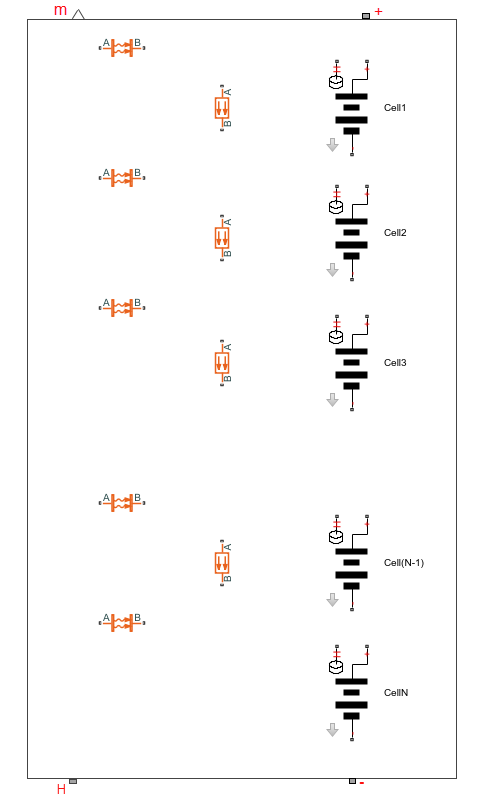### Connecting the Components

Use a `for`-loop to connect all the electrical nodes of the cells in series, by connecting the negative port of each cell (except the last one) to the positive port of the next cell:

``` for i=1:Ncells-1 connections connect(battery_cell(i+1).p,battery_cell(i).n); end end ```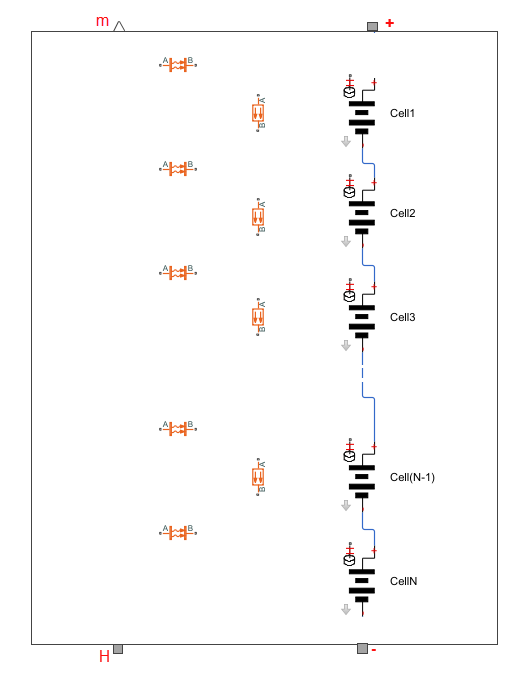Then connect this internal chain to the two electrical nodes of the composite component:

``` connections connect(battery_cell(1).p,p); connect(battery_cell(Ncells).n,n); end ```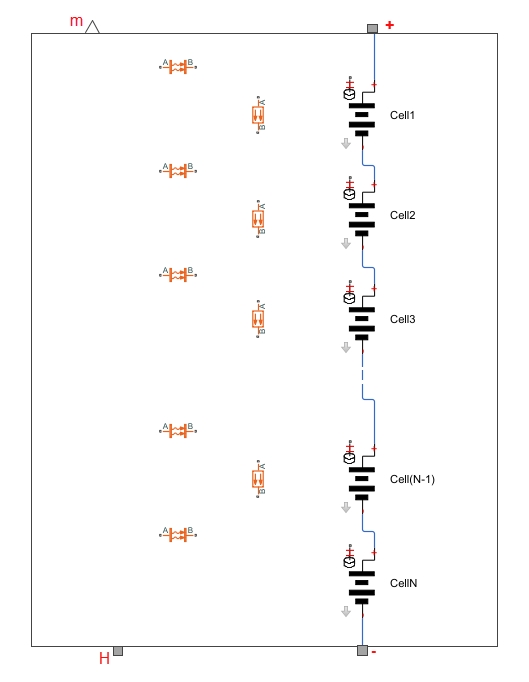Use another `for`-loop to connect thermal node `H` of each of the cells to node `B` of its respective convection component, and also to connect nodes `A` of all the convection components to thermal node `H` of the composite component:

``` for i=1:Ncells connections connect(battery_cell(i).H,convection(i).B); connect(H,convection(i).A); end end ```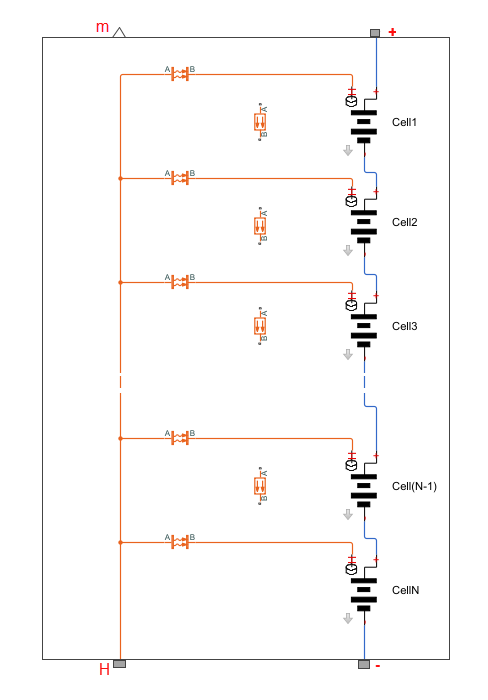Finally, incorporate the thermal conduction elements by connecting node `A` to node `H` of the previous cell and node `B` to node `H` of the next cell:

``` for i=1:Ncells-1 connections connect(battery_cell(i).H,conduction(i).A); connect(battery_cell(i+1).H,conduction(i).B); end end ```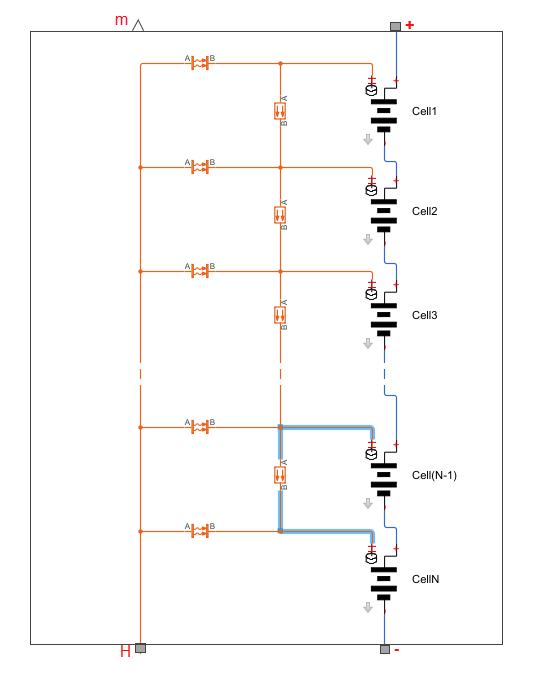### Outputting Data as a Numeric Array

The physical signal output port, `m`, outputs the temperature data for the battery pack as a multidimensional physical signal, composed of the temperature data for each cell. The vector size is determined by the `Ncells` parameter value.

```outputs m = {ones(1,Ncells),'K'}; % m:top end ```

To get the temperature data from the array of components, use the `equations` section. Declare the variable `T` as protected, because public variables cannot reference parameters, and then use this variable to extract the temperature values from the member cells.

```variables(Access=protected) T = {ones(1,Ncells),'K'}; end equations T == [battery_cell.T_init]; m == T; end ```

Indexing into member component variables, `battery_cell.T_init`, returns a comma-separated list. You can then concatenate the comma-separated list into a numeric array, `[battery_cell.T_init]`, and use it in the component equations. For more information, see How to Use Comma-Separated Lists.

### Battery Pack Block

The Battery Pack block generated from this composite component has two electrical ports, + and -, a thermal port, H, and a physical signal output port, m. The parameters modifiable through the block interface include the number of cells in the battery pack and the position of the faulted cell. The block user can modify the parameters of the faulted cell to see the impact of the fault on battery performance and cell temperatures.